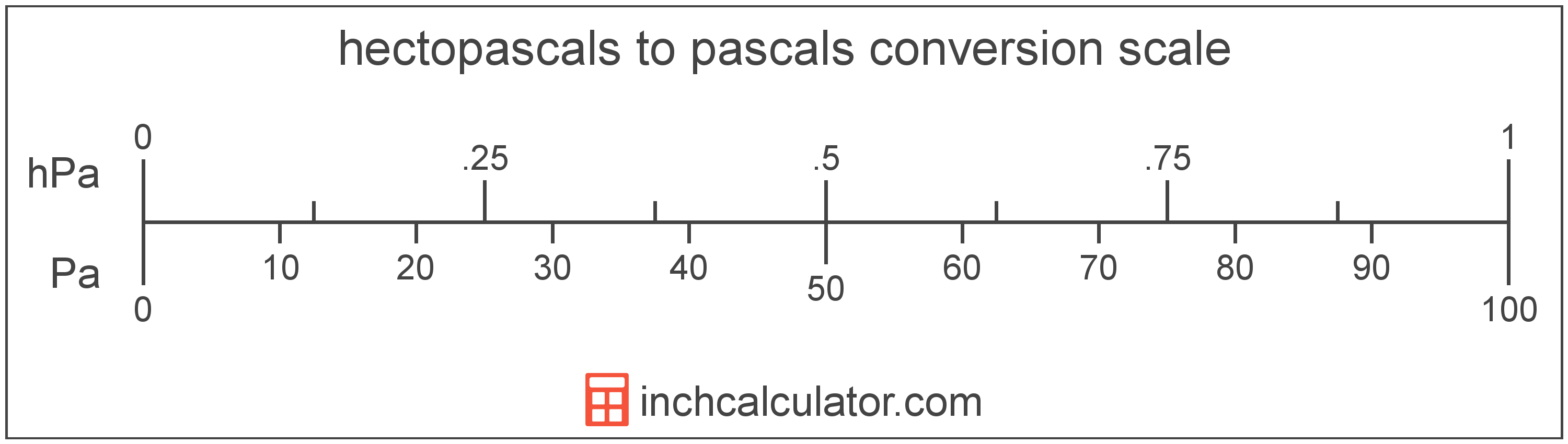# Hectopascals to Pascals Converter

Enter the pressure in hectopascals below to get the value converted to pascals.

Results in Pascals:1 hPa = 100 Pa

Do you want to convert pascals to hectopascals?

## How to Convert Hectopascals to Pascals

To convert a measurement in hectopascals to a measurement in pascals, multiply the pressure by the following conversion ratio: 100 pascals/hectopascal.

Since one hectopascal is equal to 100 pascals, you can use this simple formula to convert:

pascals = hectopascals × 100

The pressure in pascals is equal to the pressure in hectopascals multiplied by 100.

For example, here's how to convert 5 hectopascals to pascals using the formula above.
pascals = (5 hPa × 100) = 500 Pa## What Is a Hectopascal?

The hectopascal is equal to 100 pascals, which are defined as the pressure of one newton per square meter. The hectopascal is the internationally adopted unit used to measure atmospheric pressure.

The hectopascal is a multiple of the pascal, which is the SI derived unit for pressure. In the metric system, "hecto" is the prefix for 102. Hectopascals can be abbreviated as hPa; for example, 1 hectopascal can be written as 1 hPa.

## What Is a Pascal?

The pascal is defined as the pressure of one newton per square meter.

The pascal is the SI derived unit for pressure in the metric system. Pascals can be abbreviated as Pa; for example, 1 pascal can be written as 1 Pa.

Pascals can be expressed using the formula:
1 Pa = 1 N / m2

Pressure in pascals is equal to the force in newtons divided by the area in square meters.

## Hectopascal to Pascal Conversion Table

Table showing various hectopascal measurements converted to pascals.
Hectopascals Pascals
0.01 hPa 1 Pa
0.02 hPa 2 Pa
0.03 hPa 3 Pa
0.04 hPa 4 Pa
0.05 hPa 5 Pa
0.06 hPa 6 Pa
0.07 hPa 7 Pa
0.08 hPa 8 Pa
0.09 hPa 9 Pa
0.1 hPa 10 Pa
0.2 hPa 20 Pa
0.3 hPa 30 Pa
0.4 hPa 40 Pa
0.5 hPa 50 Pa
0.6 hPa 60 Pa
0.7 hPa 70 Pa
0.8 hPa 80 Pa
0.9 hPa 90 Pa
1 hPa 100 Pa